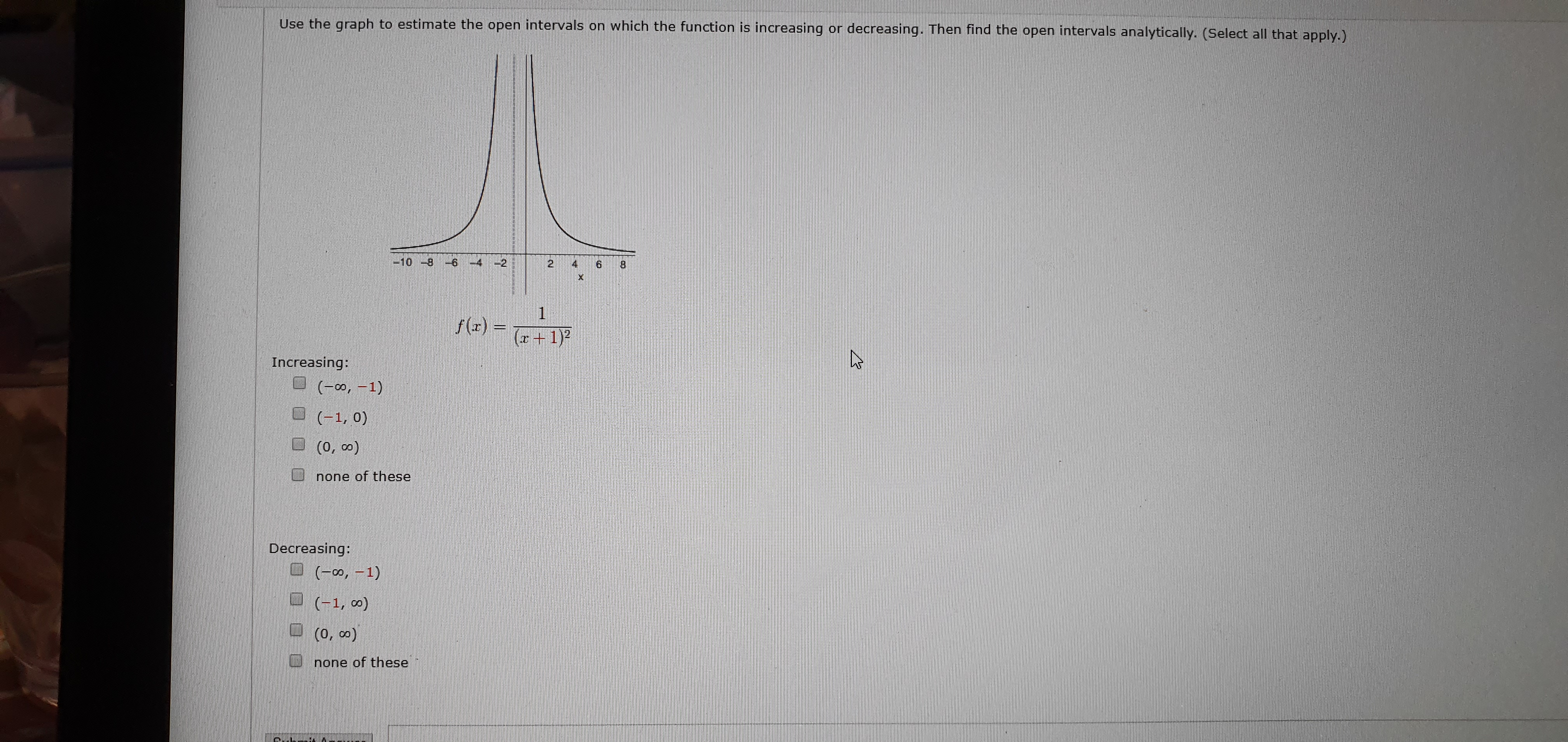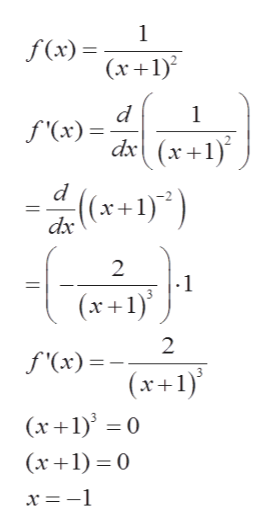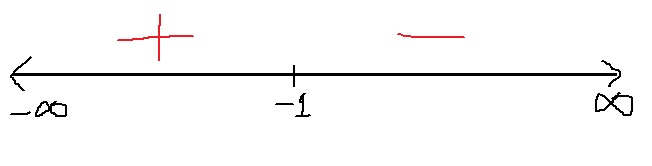# or decreasing. Then find the open intervals analytically. (Select all that apply.)Use the graph to estimate the open intervals on which the function is increasing24-10 -81f() 1Increasing:(00, 1)(1, 0)(co 0)none of theseDecreasing:(o,1)(1, co)(0, co)none of theseX

Question
210 views

Which is the result?help_outlineImage Transcriptioncloseor decreasing. Then find the open intervals analytically. (Select all that apply.) Use the graph to estimate the open intervals on which the function is increasing 2 4 -10 -8 1 f() 1 Increasing: (00, 1) (1, 0) (co 0) none of these Decreasing: (o,1) (1, co) (0, co) none of these X fullscreen
check_circle

Step 1

We are given a function as

Step 2

We can find derivative and then set top and bottom term to 0

and then we solve for xhelp_outlineImage Transcriptionclose1 f(x)x1) d 1 f'(x)= d(x+1 (x+1)) dx 2 1 (x1) 2 f'(x) (x1) (x 10 (r1)0 fullscreen
Step 3

Now, we can draw a number line

and locate x=-1

and then we can check sign of first derivative on each intervals...

### Want to see the full answer?

See Solution

#### Want to see this answer and more?

Solutions are written by subject experts who are available 24/7. Questions are typically answered within 1 hour.*

See Solution
*Response times may vary by subject and question.
Tagged in

### Calculus